StatLect

# Probability mass function

The probability mass function (pmf) characterizes the distribution of a discrete random variable. It associates to any given number the probability that the random variable will be equal to that number.## Definition

In formal terms, the probability mass function of a discrete random variableis a function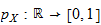such thatwhere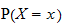is the probability that the realization of the random variablewill be equal to.

## Example

Suppose a random variablecan take only three values (1, 2 and 3), each with equal probability. Its probability mass function is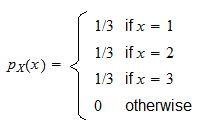So, for example,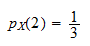that is, the probability thatwill be equal tois. Or,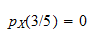that is, the probability thatwill be equal tois equal to.

## Domain

Note that the probability mass function is defined on all of, that is, it can take as argument any real number. However, its value is equal to zero for all those arguments that do not belong to the support of(i.e., to the set of values that the variablecan take). On the contrary, the value of the pmf is positive for the arguments that belong to the support of.

In the example above, the support ofis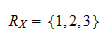As a consequence, the pmf is positive on the supportand equal to zero everywhere else.

## Plots

Often, probability mass functions are plotted as column charts. For example, the following plot shows the pmf of the Poisson distribution, which is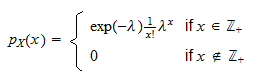We set the parameterand plot the values of the pmf only for arguments smaller than(note that the support of the distribution is, i.e., the set of all non-negative integers, but the values ofbecome very small for).

## More details

You can find an in-depth discussion of probability mass functions in the lecture entitled Random variables.

## Related concepts

Related concepts can be found in the following glossary entries:

Previous entry: Probability density function

Next entry: Probability space